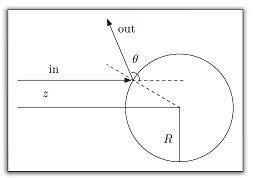# Determining Angle

## Homework Statement

Suppose a solid body approaches a sphere of radius one and height z, how do I calculate the angle ##\theta## at which the body bounces off the sphere? An image is attached:## Homework Equations

Angle of incidence, reflection and velocity

## The Attempt at a Solution

I was told that the following but I still do not know how to apply it correct.

"Break down the velocity vector of the solid body into two components: one is tangential to the sphere, another one points to the centre of the sphere. Reverse the direction of the central component and add the two component together and you will have the bounce off velocity vector. The angle between incident and reflected angle is what you need. Divide it by two and you will have the answer."

Last edited:

BvU
Homework Helper
What you were told in this thread applies if the sphere has a fixed position (i.e. is solidly welded onto whatever) and the collision is fully elastic. The one who gave you that advice forgot to mention those restrictions. I don't see any difference with the question in the current thread, so now we have a duplication, which is frowned upon as undesirable in PF.

As long as the solid body mass is much smaller than the sphere mass, both are pretty hard and neither spin nor friction play a role, it is at best a reasonable approximation.

If your application doesn't require more sophistication, then you're fine.

As to your question: there is a z in your drawing. Would that be the height above the sphere center ? Then calculate ##\pi - 2 \arctan {z\over R}##.

What you were told in this thread applies if the sphere has a fixed position (i.e. is solidly welded onto whatever) and the collision is fully elastic. The one who gave you that advice forgot to mention those restrictions. I don't see any difference with the question in the current thread, so now we have a duplication, which is frowned upon as undesirable in PF.

I am sorry, I will not do that again.

As to your question: there is a z in your drawing. Would that be the height above the sphere center ? Then calculate ##\pi - 2 \arctan {z\over R}##.

Thank you very much!